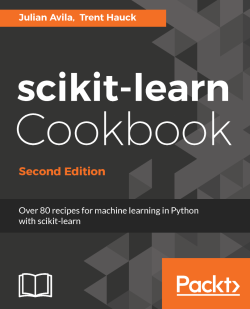# scikit-learn Cookbook - Second Edition4.7 (3 reviews total)
By Julian Avila , Trent Hauck
• \$33.99 eBook
• \$41.99 Print + eBook
• \$12.99 eBook + Subscription
What do you get with a Packt Subscription?

• Constantly updated with 100+ new titles each month
• Breadth and depth in over 1,000+ technologies

Python is quickly becoming the go-to language for analysts and data scientists due to its simplicity and flexibility, and within the Python data space, scikit-learn is the unequivocal choice for machine learning. This book includes walk throughs and solutions to the common as well as the not-so-common problems in machine learning, and how scikit-learn can be leveraged to perform various machine learning tasks effectively.

The second edition begins with taking you through recipes on evaluating the statistical properties of data and generates synthetic data for machine learning modelling. As you progress through the chapters, you will comes across recipes that will teach you to implement techniques like data pre-processing, linear regression, logistic regression, K-NN, Naïve Bayes, classification, decision trees, Ensembles and much more. Furthermore, you’ll learn to optimize your models with multi-class classification, cross validation, model evaluation and dive deeper in to implementing deep learning with scikit-learn. Along with covering the enhanced features on model section, API and new features like classifiers, regressors and estimators the book also contains recipes on evaluating and fine-tuning the performance of your model.

By the end of this book, you will have explored plethora of features offered by scikit-learn for Python to solve any machine learning problem you come across.

Publication date:
November 2017
Publisher
Packt
Pages
374
ISBN
9781787286382

# High-Performance Machine Learning – NumPy

In this chapter, we will cover the following recipes:

• NumPy basics
• Viewing the iris dataset
• Viewing the iris dataset with pandas
• Plotting with NumPy and matplotlib
• A minimal machine learning recipe – SVM classification
• Introducing cross-validation
• Putting it all together
• Machine learning overview – classification versus regression

# Introduction

In this chapter, we'll learn how to make predictions with scikit-learn. Machine learning emphasizes on measuring the ability to predict, and with scikit-learn we will predict accurately and quickly.

We will examine the iris dataset, which consists of measurements of three types of Iris flowers: Iris Setosa, Iris Versicolor, and Iris Virginica.

To measure the strength of the predictions, we will:

• Save some data for testing
• Build a model using only training data
• Measure the predictive power on the test set

The prediction—one of three flower types is categorical. This type of problem is called a classification problem.

Informally, classification asks, Is it an apple or an orange? Contrast this with machine learning regression, which asks, How many apples? By the way, the answer can be 4.5 apples for regression.

By the evolution of its design, scikit-learn addresses machine learning mainly via four categories:

• Classification:
• Non-text classification, like the Iris flowers example
• Text classification
• Regression
• Clustering
• Dimensionality reduction

# NumPy basics

Data science deals in part with structured tables of data. The scikit-learn library requires input tables of two-dimensional NumPy arrays. In this section, you will learn about the numpy library.

# How to do it...

We will try a few operations on NumPy arrays. NumPy arrays have a single type for all of their elements and a predefined shape. Let us look first at their shape.

# The shape and dimension of NumPy arrays

1. Start by importing NumPy:
`import numpy as np`
1. Produce a NumPy array of 10 digits, similar to Python's range(10) method:
`np.arange(10)array([0, 1, 2, 3, 4, 5, 6, 7, 8, 9])`
1. The array looks like a Python list with only one pair of brackets. This means it is of one dimension. Store the array and find out the shape:
`array_1 = np.arange(10)array_1.shape(10L,)`
1. The array has a data attribute, shape. The type of array_1.shape is a tuple (10L,), which has length 1, in this case. The number of dimensions is the same as the length of the tuple—a dimension of 1, in this case:
`array_1.ndim      #Find number of dimensions of array_11`
1. The array has 10 elements. Reshape the array by calling the reshape method:
`array_1.reshape((5,2))array([[0, 1],       [2, 3],       [4, 5],       [6, 7],       [8, 9]])`
1. This reshapes the array into 5 x 2 data object that resembles a list of lists (a three dimensional NumPy array looks like a list of lists of lists). You did not save the changes. Save the reshaped array as follows::
`array_1 = array_1.reshape((5,2))`
1. Note that array_1 is now two-dimensional. This is expected, as its shape has two numbers and it looks like a Python list of lists:
`array_1.ndim2`

1. Add 1 to every element of the array by broadcasting. Note that changes to the array are not saved:
`array_1 + 1array([[ 1,  2],       [ 3,  4],       [ 5,  6],       [ 7,  8],       [ 9, 10]])`

The term broadcasting refers to the smaller array being stretched or broadcast across the larger array. In the first example, the scalar 1 was stretched to a 5 x 2 shape and then added to array_1.

1. Create a new array_2 array. Observe what occurs when you multiply the array by itself (this is not matrix multiplication; it is element-wise multiplication of arrays):
`array_2 = np.arange(10)array_2 * array_2array([ 0,  1,  4,  9, 16, 25, 36, 49, 64, 81])`
1. Every element has been squared. Here, element-wise multiplication has occurred. Here is a more complicated example:
`array_2 = array_2 ** 2  #Note that this is equivalent to array_2 * array_2array_2 = array_2.reshape((5,2))array_2array([[ 0,  1],       [ 4,  9],       [16, 25],       [36, 49],       [64, 81]])`
1. Change array_1 as well:
`array_1 = array_1 + 1array_1array([[ 1,  2],       [ 3,  4],       [ 5,  6],       [ 7,  8],       [ 9, 10]])`
1. Now add array_1 and array_2 element-wise by simply placing a plus sign between the arrays:
`array_1 + array_2array([[ 1,  3],       [ 7, 13],       [21, 31],       [43, 57],       [73, 91]])`
1. The formal broadcasting rules require that whenever you are comparing the shapes of both arrays from right to left, all the numbers have to either match or be one. The shapes 5 X 2 and 5 X 2 match for both entries from right to left. However, the shape 5 X 2 X 1 does not match 5 X 2, as the second values from the right, 2 and 5 respectively, are mismatched: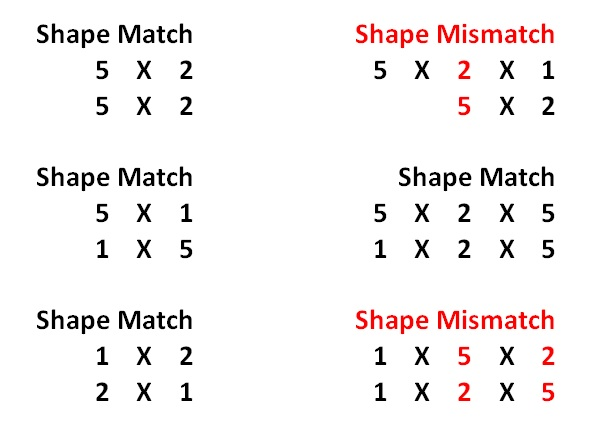# Initializing NumPy arrays and dtypes

There are several ways to initialize NumPy arrays besides np.arange:

1. Initialize an array of zeros with np.zeros. The np.zeros((5,2)) command creates a 5 x 2 array of zeros:
`np.zeros((5,2))array([[ 0.,  0.],       [ 0.,  0.],       [ 0.,  0.],       [ 0.,  0.],       [ 0.,  0.]])`
1. Initialize an array of ones using np.ones. Introduce a dtype argument, set to np.int, to ensure that the ones are of NumPy integer type. Note that scikit-learn expects np.float arguments in arrays. The dtype refers to the type of every element in a NumPy array. It remains the same throughout the array. Every single element of the array below has a np.int integer type.
`np.ones((5,2), dtype = np.int)array([[1, 1],       [1, 1],       [1, 1],       [1, 1],       [1, 1]])`
1. Use np.empty to allocate memory for an array of a specific size and dtype, but no particular initialized values:
`np.empty((5,2), dtype = np.float)array([[  3.14724935e-316,   3.14859499e-316],       [  3.14858945e-316,   3.14861159e-316],       [  3.14861435e-316,   3.14861712e-316],       [  3.14861989e-316,   3.14862265e-316],       [  3.14862542e-316,   3.14862819e-316]])`
1. Use np.zeros, np.ones, and np.empty to allocate memory for NumPy arrays with different initial values.

# Indexing

1. Look up the values of the two-dimensional arrays with indexing:
`array_1[0,0]   #Finds value in first row and first column.1`
1. View the first row:
`array_1[0,:]array([1, 2])`
1. Then view the first column:
`array_1[:,0]array([1, 3, 5, 7, 9])`
1. View specific values along both axes. Also view the second to the fourth rows:
`array_1[2:5, :]array([[ 5,  6],       [ 7,  8],       [ 9, 10]])`
1. View the second to the fourth rows only along the first column:
`array_1[2:5,0]array([5, 7, 9])`

# Boolean arrays

Additionally, NumPy handles indexing with Boolean logic:

1. First produce a Boolean array:
`array_1 > 5array([[False, False],       [False, False],       [False,  True],       [ True,  True],       [ True,  True]], dtype=bool)`
1. Place brackets around the Boolean array to filter by the Boolean array:
`array_1[array_1 > 5]array([ 6,  7,  8,  9, 10])`

# Arithmetic operations

1. Add all the elements of the array with the sum method. Go back to array_1:
`array_1array([[ 1,  2],       [ 3,  4],       [ 5,  6],       [ 7,  8],       [ 9, 10]])array_1.sum()55`
1. Find all the sums by row:
`array_1.sum(axis = 1)array([ 3,  7, 11, 15, 19])`
1. Find all the sums by column:
`array_1.sum(axis = 0)array([25, 30])`
1. Find the mean of each column in a similar way. Note that the dtype of the array of averages is np.float:
`array_1.mean(axis = 0)array([ 5.,  6.])`

# NaN values

1. Scikit-learn will not accept np.nan values. Take array_3 as follows:
`array_3 = np.array([np.nan, 0, 1, 2, np.nan])`
1. Find the NaN values with a special Boolean array created by the np.isnan function:
`np.isnan(array_3)array([ True, False, False, False,  True], dtype=bool)`
1. Filter the NaN values by negating the Boolean array with the symbol ~ and placing brackets around the expression:
`array_3[~np.isnan(array_3)]>array([ 0.,  1.,  2.])`
1. Alternatively, set the NaN values to zero:
`array_3[np.isnan(array_3)] = 0array_3array([ 0.,  0.,  1.,  2.,  0.])`

# How it works...

Data, in the present and minimal sense, is about 2D tables of numbers, which NumPy handles very well. Keep this in mind in case you forget the NumPy syntax specifics. Scikit-learn accepts only 2D NumPy arrays of real numbers with no missing np.nan values.

From experience, it tends to be best to change np.nan to some value instead of throwing away data. Personally, I like to keep track of Boolean masks and keep the data shape roughly the same, as this leads to fewer coding errors and more coding flexibility.

To perform machine learning with scikit-learn, we need some data to start with. We will load the iris dataset, one of the several datasets available in scikit-learn.

A scikit-learn program begins with several imports. Within Python, preferably in Jupyter Notebook, load the numpy, pandas, and pyplot libraries:

`import numpy as np    #Load the numpy library for fast array computationsimport pandas as pd   #Load the pandas data-analysis libraryimport matplotlib.pyplot as plt   #Load the pyplot visualization library`

If you are within a Jupyter Notebook, type the following to see a graphical output instantly:

`%matplotlib inline `

# How to do it...

1. From the scikit-learn datasets module, access the iris dataset:
`from sklearn import datasetsiris = datasets.load_iris()`

# How it works...

Similarly, you could have imported the diabetes dataset as follows:

`from sklearn import datasets  #Import datasets module from scikit-learndiabetes = datasets.load_diabetes()   `

There! You've loaded diabetes using the load_diabetes() function of the datasets module. To check which datasets are available, type:

`datasets.load_*?`

Once you try that, you might observe that there is a dataset named datasets.load_digits. To access it, type the load_digits() function, analogous to the other loading functions:

`digits = datasets.load_digits()`

To view information about the dataset, type digits.DESCR.

# Viewing the iris dataset

Now that we've loaded the dataset, let's examine what is in it. The iris dataset pertains to a supervised classification problem.

# How to do it...

1. To access the observation variables, type:
`iris.data`

This outputs a NumPy array:

`array([[ 5.1,  3.5,  1.4,  0.2],       [ 4.9,  3. ,  1.4,  0.2],       [ 4.7,  3.2,  1.3,  0.2], #...rest of output suppressed because of length`
1. Let's examine the NumPy array:
`iris.data.shape`

This returns:

`(150L, 4L)`

This means that the data is 150 rows by 4 columns. Let's look at the first row:

`iris.dataarray([ 5.1,  3.5,  1.4,  0.2])`

The NumPy array for the first row has four numbers.

1. To determine what they mean, type:
`iris.feature_names['sepal length (cm)', 'sepal width (cm)', 'petal length (cm)', 'petal width (cm)']`

The feature or column names name the data. They are strings, and in this case, they correspond to dimensions in different types of flowers. Putting it all together, we have 150 examples of flowers with four measurements per flower in centimeters. For example, the first flower has measurements of 5.1 cm for sepal length, 3.5 cm for sepal width, 1.4 cm for petal length, and 0.2 cm for petal width. Now, let's look at the output variable in a similar manner:

`iris.target`

This yields an array of outputs: 0, 1, and 2. There are only three outputs. Type this:

`iris.target.shape`

You get a shape of:

`(150L,)`

This refers to an array of length 150 (150 x 1). Let's look at what the numbers refer to:

`iris.target_namesarray(['setosa', 'versicolor', 'virginica'],       dtype='|S10')`

The output of the iris.target_names variable gives the English names for the numbers in the iris.target variable. The number zero corresponds to the setosa flower, number one corresponds to the versicolor flower, and number two corresponds to the virginica flower. Look at the first row of iris.target:

`iris.target`

This produces zero, and thus the first row of observations we examined before correspond to the setosa flower.

# How it works...

In machine learning, we often deal with data tables and two-dimensional arrays corresponding to examples. In the iris set, we have 150 observations of flowers of three types. With new observations, we would like to predict which type of flower those observations correspond to. The observations in this case are measurements in centimeters. It is important to look at the data pertaining to real objects. Quoting my high school physics teacher, "Do not forget the units!"

The iris dataset is intended to be for a supervised machine learning task because it has a target array, which is the variable we desire to predict from the observation variables. Additionally, it is a classification problem, as there are three numbers we can predict from the observations, one for each type of flower. In a classification problem, we are trying to distinguish between categories. The simplest case is binary classification. The iris dataset, with three flower categories, is a multi-class classification problem.

# There's more...

With the same data, we can rephrase the problem in many ways, or formulate new problems. What if we want to determine relationships between the observations? We can define the petal width as the target variable. We can rephrase the problem as a regression problem and try to predict the target variable as a real number, not just three categories. Fundamentally, it comes down to what we intend to predict. Here, we desire to predict a type of flower.

# Viewing the iris dataset with Pandas

In this recipe we will use the handy pandas data analysis library to view and visualize the iris dataset. It contains the notion o, a dataframe which might be familiar to you if you use the language R's dataframe.

# How to do it...

You can view the iris dataset with Pandas, a library built on top of NumPy:

1. Create a dataframe with the observation variables iris.data, and column names columns, as arguments:
`import pandas as pdiris_df = pd.DataFrame(iris.data, columns = iris.feature_names)`

The dataframe is more user-friendly than the NumPy array.

1. Look at a quick histogram of the values in the dataframe for sepal length:
`iris_df['sepal length (cm)'].hist(bins=30)`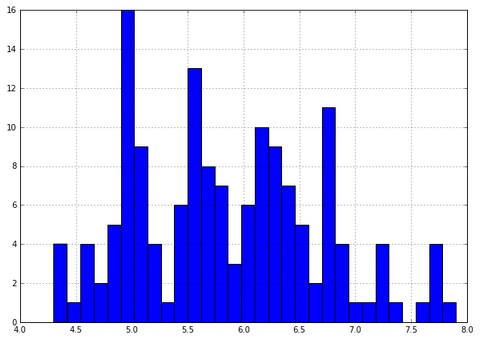1. You can also color the histogram by the target variable:
`for class_number in np.unique(iris.target):    plt.figure(1)    iris_df['sepal length (cm)'].iloc[np.where(iris.target == class_number)].hist(bins=30)`
1. Here, iterate through the target numbers for each flower and draw a color histogram for each. Consider this line:
`np.where(iris.target== class_number)`

It finds the NumPy index location for each class of flower: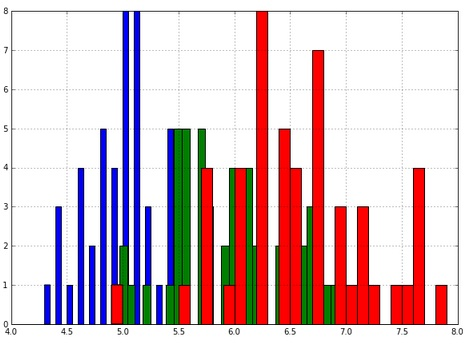Observe that the histograms overlap. This encourages us to model the three histograms as three normal distributions. This is possible in a machine learning manner if we model the training data only as three normal distributions, not the whole set. Then we use the test set to test the three normal distribution models we just made up. Finally, we test the accuracy of our predictions on the test set.

# How it works...

The dataframe data object is a 2D NumPy array with column names and row names. In data science, the fundamental data object looks like a 2D table, possibly because of SQL's long history. NumPy allows for 3D arrays, cubes, 4D arrays, and so on. These also come up often.

# Plotting with NumPy and matplotlib

A simple way to make visualizations with NumPy is by using the library matplotlib. Let's make some visualizations quickly.

Start by importing numpy and matplotlib. You can view visualizations within an IPython Notebook using the %matplotlib inline command:

`import numpy as np import matplotlib.pyplot as plt %matplotlib inline`

# How to do it...

1. The main command in matplotlib, in pseudo code, is as follows:
`plt.plot(numpy array, numpy array of same length)`
1. Plot a straight line by placing two NumPy arrays of the same length:
`plt.plot(np.arange(10), np.arange(10))`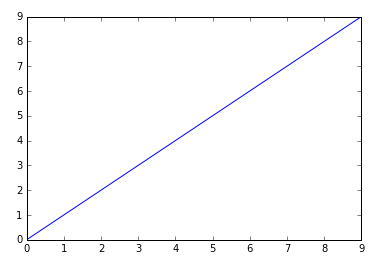1. Plot an exponential:
`plt.plot(np.arange(10), np.exp(np.arange(10)))`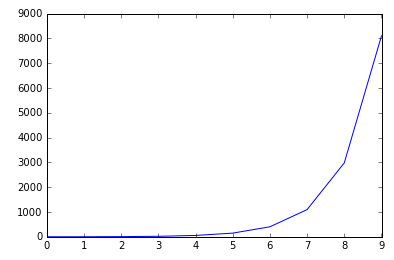1. Place the two graphs side by side:
`plt.figure()plt.subplot(121)plt.plot(np.arange(10), np.exp(np.arange(10)))plt.subplot(122)plt.scatter(np.arange(10), np.exp(np.arange(10)))`

Or top to bottom:

`plt.figure()plt.subplot(211)plt.plot(np.arange(10), np.exp(np.arange(10)))plt.subplot(212)plt.scatter(np.arange(10), np.exp(np.arange(10)))`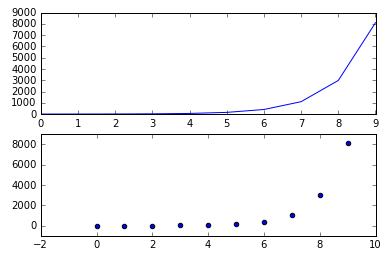The first two numbers in the subplot command refer to the grid size in the figure instantiated by plt.figure(). The grid size referred to in plt.subplot(221) is 2 x 2, the first two digits. The last digit refers to traversing the grid in reading order: left to right and then up to down.

1. Plot in a 2 x 2 grid traversing in reading order from one to four:
`plt.figure()plt.subplot(221)plt.plot(np.arange(10), np.exp(np.arange(10)))plt.subplot(222)plt.scatter(np.arange(10), np.exp(np.arange(10)))plt.subplot(223)plt.scatter(np.arange(10), np.exp(np.arange(10)))plt.subplot(224)plt.scatter(np.arange(10), np.exp(np.arange(10)))`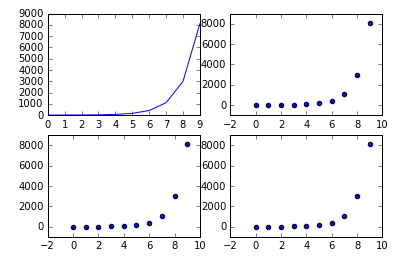1. Finally, with real data:
`from sklearn.datasets import load_irisiris = load_iris()data = iris.datatarget = iris.target# Resize the figure for better viewingplt.figure(figsize=(12,5))# First subplotplt.subplot(121)# Visualize the first two columns of data:plt.scatter(data[:,0], data[:,1], c=target)# Second subplotplt.subplot(122)# Visualize the last two columns of data:plt.scatter(data[:,2], data[:,3], c=target)`

The c parameter takes an array of colors—in this case, the colors 0, 1, and 2 in the iris target: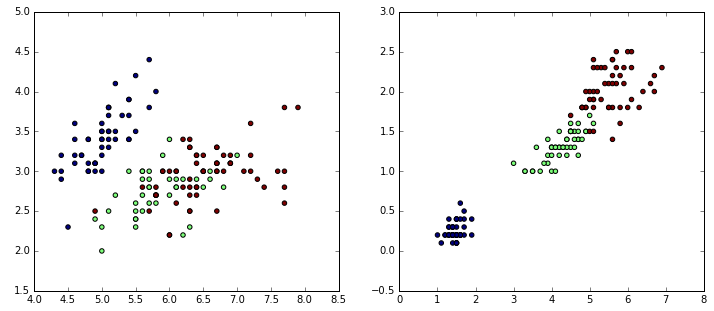# A minimal machine learning recipe – SVM classification

Machine learning is all about making predictions. To make predictions, we will:

• State the problem to be solved
• Choose a model to solve the problem
• Train the model
• Make predictions
• Measure how well the model performed

Back to the iris example, we now store the first two features (columns) of the observations as X and the target as y, a convention in the machine learning community:

`X = iris.data[:, :2]  y = iris.target`

# How to do it...

1. First, we state the problem. We are trying to determine the flower-type category from a set of new observations. This is a classification task. The data available includes a target variable, which we have named y. This is a supervised classification problem.
The task of supervised learning involves predicting values of an output variable with a model that trains using input variables and an output variable.
1. Next, we choose a model to solve the supervised classification. For now, we will use a support vector classifier. Because of its simplicity and interpretability, it is a commonly used algorithm (interpretable means easy to read into and understand).
2. To measure the performance of prediction, we will split the dataset into training and test sets. The training set refers to data we will learn from. The test set is the data we hold out and pretend not to know as we would like to measure the performance of our learning procedure. So, import a function that will split the dataset:
`from sklearn.model_selection import train_test_split`
1. Apply the function to both the observation and target data:

`X_train, X_test, y_train, y_test = train_test_split(X, y, test_size=0.25, random_state=1)`

The test size is 0.25 or 25% of the whole dataset. A random state of one fixes the random seed of the function so that you get the same results every time you call the function, which is important for now to reproduce the same results consistently.

1. Now load a regularly used estimator, a support vector machine:
`from sklearn.svm import SVC`
1. You have imported a support vector classifier from the svm module. Now create an instance of a linear SVC:
`clf = SVC(kernel='linear',random_state=1)`

The random state is fixed to reproduce the same results with the same code later.

The supervised models in scikit-learn implement a fit(X, y) method, which trains the model and returns the trained model. X is a subset of the observations, and each element of y corresponds to the target of each observation in X. Here, we fit a model on the training data:

`clf.fit(X_train, y_train)`

Now, the clf variable is the fitted, or trained, model.

The estimator also has a predict(X) method that returns predictions for several unlabeled observations, X_test, and returns the predicted values, y_pred. Note that the function does not return the estimator. It returns a set of predictions:

`y_pred = clf.predict(X_test)`

So far, you have done all but the last step. To examine the model performance, load a scorer from the metrics module:

`from sklearn.metrics import accuracy_score`

With the scorer, compare the predictions with the held-out test targets:

`accuracy_score(y_test,y_pred)0.76315789473684215`

# How it works...

Without knowing very much about the details of support vector machines, we have implemented a predictive model. To perform machine learning, we held out one-fourth of the data and examined how the SVC performed on that data. In the end, we obtained a number that measures accuracy, or how the model performed.

# There's more...

To summarize, we will do all the steps with a different algorithm, logistic regression:

1. First, import LogisticRegression:
`from sklearn.linear_model import LogisticRegression`
1. Then write a program with the modeling steps:
1. Split the data into training and testing sets.
2. Fit the logistic regression model.
3. Predict using the test observations.
4. Measure the accuracy of the predictions with y_test versus y_pred:
`import matplotlib.pyplot as pltfrom sklearn import datasetsfrom sklearn.model_selection import train_test_splitfrom sklearn.metrics import accuracy_scoreX = iris.data[:, :2]   #load the iris datay = iris.targetX_train, X_test, y_train, y_test = train_test_split(X, y, test_size=0.25, random_state=1)#train the modelclf = LogisticRegression(random_state = 1)clf.fit(X_train, y_train)#predict with Logistic Regressiony_pred = clf.predict(X_test)#examine the model accuracyaccuracy_score(y_test,y_pred)0.60526315789473684`

This number is lower; yet we cannot make any conclusions comparing the two models, SVC and logistic regression classification. We cannot compare them, because we were not supposed to look at the test set for our model. If we made a choice between SVC and logistic regression, the choice would be part of our model as well, so the test set cannot be involved in the choice. Cross-validation, which we will look at next, is a way to choose between models.

# Introducing cross-validation

We are thankful for the iris dataset, but as you might recall, it has only 150 observations. To make the most out of the set, we will employ cross-validation. Additionally, in the last section, we wanted to compare the performance of two different classifiers, support vector classifier and logistic regression. Cross-validation will help us with this comparison issue as well.

Suppose we wanted to choose between the support vector classifier and the logistic regression classifier. We cannot measure their performance on the unavailable test set.

• Forgot about the test set for now?
• Split the training set into two parts, one to train on and one to test the training?

Split the training set into two parts using the train_test_split function used in previous sections:

`from sklearn.model_selection import train_test_splitX_train_2, X_test_2, y_train_2, y_test_2 = train_test_split(X_train, y_train, test_size=0.25, random_state=1)`

X_train_2 consists of 75% of the X_train data, while X_test_2 is the remaining 25%. y_train_2 is 75% of the target data, and matches the observations of X_train_2. y_test_2 is 25% of the target data present in y_train.

As you might have expected, you have to use these new splits to choose between the two models: SVC and logistic regression. Do so by writing a predictive program.

# How to do it...

`from sklearn import datasetsfrom sklearn.model_selection import train_test_splitfrom sklearn.metrics import accuracy_score#load the classifying modelsfrom sklearn.linear_model import LogisticRegressionfrom sklearn.svm import SVCiris = datasets.load_iris()X = iris.data[:, :2]  #load the first two features of the iris data y = iris.target #load the target of the iris data#split the whole set one time#Note random state is 7 nowX_train, X_test, y_train, y_test = train_test_split(X, y, test_size=0.25, random_state=7)#split the training set into partsX_train_2, X_test_2, y_train_2, y_test_2 = train_test_split(X_train, y_train, test_size=0.25, random_state=7)`
1. Create an instance of an SVC classifier and fit it:
`svc_clf = SVC(kernel = 'linear',random_state = 7)svc_clf.fit(X_train_2, y_train_2)`
1. Do the same for logistic regression (both lines for logistic regression are compressed into one):
`lr_clf = LogisticRegression(random_state = 7).fit(X_train_2, y_train_2)`
1. Now predict and examine the SVC and logistic regression's performance on X_test_2:
`svc_pred = svc_clf.predict(X_test_2)lr_pred = lr_clf.predict(X_test_2)print "Accuracy of SVC:",accuracy_score(y_test_2,svc_pred)print "Accuracy of LR:",accuracy_score(y_test_2,lr_pred)Accuracy of SVC: 0.857142857143Accuracy of LR: 0.714285714286`
1. The SVC performs better, but we have not yet seen the original test data. Choose SVC over logistic regression and try it on the original test set:
`print "Accuracy of SVC on original Test Set: ",accuracy_score(y_test, svc_clf.predict(X_test))Accuracy of SVC on original Test Set:  0.684210526316`

# How it works...

In comparing the SVC and logistic regression classifier, you might wonder (and be a little suspicious) about a lot of scores being very different. The final test on SVC scored lower than logistic regression. To help with this situation, we can do cross-validation in scikit-learn.

Cross-validation involves splitting the training set into parts, as we did before. To match the preceding example, we split the training set into four parts, or folds. We are going to design a cross-validation iteration by taking turns with one of the four folds for testing and the other three for training. It is the same split as done before four times over with the same set, thereby rotating, in a sense, the test set: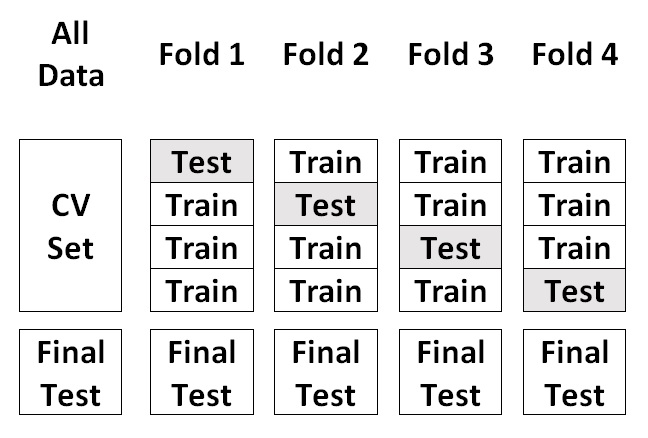With scikit-learn, this is relatively easy to accomplish:

`from sklearn.model_selection import cross_val_score`
1. Then we produce an accuracy score on four folds:
`svc_scores = cross_val_score(svc_clf, X_train, y_train, cv=4)svc_scoresarray([ 0.82758621,  0.85714286,  0.92857143,  0.77777778])`
1. We can find the mean for average performance and standard deviation for a measure of spread of all scores relative to the mean:
`print "Average SVC scores: ", svc_scores.mean()print "Standard Deviation of SVC scores: ", svc_scores.std()Average SVC scores:  0.847769567597Standard Deviation of SVC scores:  0.0545962864696`
1. Similarly, with the logistic regression instance, we compute four scores:
`lr_scores = cross_val_score(lr_clf, X_train, y_train, cv=4)print "Average SVC scores: ", lr_scores.mean()print "Standard Deviation of SVC scores: ", lr_scores.std()Average SVC scores:  0.748893906221Standard Deviation of SVC scores:  0.0485633168699`

Now we have many scores, which confirms our selection of SVC over logistic regression. Thanks to cross-validation, we used the training multiple times and had four small test sets within it to score our model.

Note that our model is a bigger model that consists of:

• Training an SVM through cross-validation
• Training a logistic regression through cross-validation
• Choosing between SVM and logistic regression
The choice at the end is part of the model.

# There's more...

Despite our hard work and the elegance of the scikit-learn syntax, the score on the test set at the very end remains suspicious. The reason for this is that the test and train split are not necessarily balanced; the train and test sets do not necessarily have similar proportions of all the classes.

This is easily remedied by using a stratified test-train split:

`X_train, X_test, y_train, y_test = train_test_split(X, y, stratify=y)`

By selecting the target set as the stratified argument, the target classes are balanced. This brings the SVC scores closer together.

`svc_scores = cross_val_score(svc_clf, X_train, y_train, cv=4)print "Average SVC scores: " , svc_scores.mean()print "Standard Deviation of SVC scores: ", svc_scores.std()print "Score on Final Test Set:", accuracy_score(y_test, svc_clf.predict(X_test))Average SVC scores:  0.831547619048Standard Deviation of SVC scores:  0.0792488953372Score on Final Test Set: 0.789473684211`

Additionally, note that in the preceding example, the cross-validation procedure produces stratified folds by default:

`from sklearn.model_selection import cross_val_scoresvc_scores = cross_val_score(svc_clf, X_train, y_train, cv = 4)`

The preceding code is equivalent to:

`from sklearn.model_selection import cross_val_score, StratifiedKFoldskf = StratifiedKFold(n_splits = 4)svc_scores = cross_val_score(svc_clf, X_train, y_train, cv = skf)`

# Putting it all together

Now, we are going to perform the same procedure as before, except that we will reset, regroup, and try a new algorithm: K-Nearest Neighbors (KNN).

# How to do it...

1. Start by importing the model from sklearn, followed by a balanced split:
`from sklearn.neighbors import KNeighborsClassifierX_train, X_test, y_train, y_test = train_test_split(X, y, stratify=y, random_state = 0)`
The random_state parameter fixes the random_seed in the function train_test_split. In the preceding example, the random_state is set to zero and can be set to any integer.
1. Construct two different KNN models by varying the n_neighbors parameter. Observe that the number of folds is now 10. Tenfold cross-validation is common in the machine learning community, particularly in data science competitions:
`from sklearn.model_selection import cross_val_scoreknn_3_clf = KNeighborsClassifier(n_neighbors = 3)knn_5_clf = KNeighborsClassifier(n_neighbors = 5)knn_3_scores = cross_val_score(knn_3_clf, X_train, y_train, cv=10)knn_5_scores = cross_val_score(knn_5_clf, X_train, y_train, cv=10)`
1. Score and print out the scores for selection:
`print "knn_3 mean scores: ", knn_3_scores.mean(), "knn_3 std: ",knn_3_scores.std()print "knn_5 mean scores: ", knn_5_scores.mean(), " knn_5 std: ",knn_5_scores.std()knn_3 mean scores:  0.798333333333 knn_3 std:  0.0908142181722knn_5 mean scores:  0.806666666667 knn_5 std:  0.0559320575496`

Both nearest neighbor types score similarly, yet the KNN with parameter n_neighbors = 5 is a bit more stable. This is an example of hyperparameter optimization which we will examine closely throughout the book.

# There's more...

You could have just as easily run a simple loop to score the function more quickly:

`all_scores = []for n_neighbors in range(3,9,1):    knn_clf = KNeighborsClassifier(n_neighbors = n_neighbors)    all_scores.append((n_neighbors, cross_val_score(knn_clf, X_train, y_train, cv=10).mean()))sorted(all_scores, key = lambda x:x, reverse = True)  `

Its output suggests that n_neighbors = 4 is a good choice:

`[(4, 0.85111111111111115), (7, 0.82611111111111113), (6, 0.82333333333333347), (5, 0.80666666666666664), (3, 0.79833333333333334), (8, 0.79833333333333334)]`

# Machine learning overview – classification versus regression

In this recipe we will examine how regression can be viewed as being very similar to classification. This is done by reconsidering the categorical labels of regression as real numbers. In this section we will also look at at several aspects of machine learning from a very broad perspective including the purpose of scikit-learn. scikit-learn allows us to find models that work well incredibly quickly. We do not have to work out all the details of the model, or optimize, until we found one that works well. Consequently, your company saves precious development time and computational resources thanks to scikit-learn giving us the ability to develop models relatively quickly.

# The purpose of scikit-learn

As we have seen before, scikit-learn allowed us to find a model that works fairly quickly. We tried SVC, logistic regression, and a few KNN classifiers. Through cross-validation, we selected models that performed better than others. In industry, after trying SVMs and logistic regression, we might focus on SVMs and optimize them further. Thanks to scikit-learn, we saved a lot of time and resources, including mental energy. After optimizing the SVM at work on a realistic dataset, we might re-implement it for speed in Java or C and gather more data.

# Supervised versus unsupervised

Classification and regression are supervised, as we know the target variables for the observations. Clustering—creating regions in space for each category without being given any labels is unsupervised learning.

In classification, the target variable is one of several categories, and there must be more than one instance of every category. In regression, there can be only one instance of every target variable, as the only requirement is that the target is a real number.

In the case of logistic regression, we saw previously that the algorithm first performs a regression and estimates a real number for the target. Then the target class is estimated by using thresholds. In scikit-learn, there are predict_proba methods that yield probabilistic estimates, which relate regression-like real number estimates with classification classes in the style of logistic regression.

Any regression can be turned into classification by using thresholds. A binary classification can be viewed as a regression problem by using a regressor. The target variables produced will be real numbers, not the original class variables.

# Quick SVC – a classifier and regressor

1. Load iris from the datasets module:
`import numpy as npimport pandas as pdfrom sklearn import datasetsiris = datasets.load_iris()`
1. For simplicity, consider only targets 0 and 1, corresponding to Setosa and Versicolor. Use the Boolean array iris.target < 2 to filter out target 2. Place it within brackets to use it as a filter in defining the observation set X and the target set y:
`X = iris.data[iris.target < 2]y = iris.target[iris.target < 2]`
1. Now import train_test_split and apply it:
`from sklearn.model_selection import train_test_splitfrom sklearn.metrics import accuracy_scoreX_train, X_test, y_train, y_test = train_test_split(X, y, stratify=y, random_state= 7)`
1. Prepare and run an SVC by importing it and scoring it with cross-validation:
`from sklearn.svm import SVCfrom sklearn.model_selection import cross_val_scoresvc_clf = SVC(kernel = 'linear').fit(X_train, y_train)svc_scores = cross_val_score(svc_clf, X_train, y_train, cv=4)`
1. As done in previous sections, view the average of the scores:
`svc_scores.mean()0.94795321637426899`
1. Perform the same with support vector regression by importing SVR from sklearn.svm, the same module that contains SVC:
`from sklearn.svm import SVR`
1. Then write the necessary syntax to fit the model. It is almost identical to the syntax for SVC, just replacing some c keywords with r:
`svr_clf = SVR(kernel = 'linear').fit(X_train, y_train)`

# Making a scorer

To make a scorer, you need:

• A scoring function that compares y_test, the ground truth, with y_pred, the predictions
• To determine whether a high score is good or bad

Before passing the SVR regressor to the cross-validation, make a scorer by supplying two elements:

1. In practice, begin by importing the make_scorer function:
`from sklearn.metrics import make_scorer`
1. Use this sample scoring function:
`#Only works for this iris example with targets 0 and 1def for_scorer(y_test, orig_y_pred):    y_pred = np.rint(orig_y_pred).astype(np.int)   #rounds prediction to the nearest integer    return accuracy_score(y_test, y_pred)`

The np.rint function rounds off the prediction to the nearest integer, hopefully one of the targets, 0 or 1. The astype method changes the type of the prediction to integer type, as the original target is in integer type and consistency is preferred with regard to types. After the rounding occurs, the scoring function uses the old accuracy_score function, which you are familiar with.

1. Now, determine whether a higher score is better. Higher accuracy is better, so for this situation, a higher score is better. In scikit code:
`svr_to_class_scorer = make_scorer(for_scorer, greater_is_better=True) `
1. Finally, run the cross-validation with a new parameter, the scoring parameter:
`svr_scores = cross_val_score(svr_clf, X_train, y_train, cv=4, scoring = svr_to_class_scorer)`
1. Find the mean:
`svr_scores.mean()0.94663742690058483`

The accuracy scores are similar for the SVR regressor-based classifier and the traditional SVC classifier.

# How it works...

You might ask, why did we take out class 2 out of the target set?

The reason is that, to use a regressor, our intent has to be to predict a real number. The categories had to have real number properties: that they are ordered (informally, if we have three ordered categories x, y, z and x < y and y < z then x < z). By eliminating the third category, the remaining flowers (Setosa and Versicolor) became ordered by a property we invented: Setosaness or Versicolorness.

The next time you encounter categories, you can consider whether they can be ordered. For example, if the dataset consists of shoe sizes, they can be ordered and a regressor can be applied, even though no one has a shoe size of 12.125.

# Linear versus nonlinear

Linear algorithms involve lines or hyperplanes. Hyperplanes are flat surfaces in any n-dimensional space. They tend to be easy to understand and explain, as they involve ratios (with an offset). Some functions that consistently and monotonically increase or decrease can be mapped to a linear function with a transformation. For example, exponential growth can be mapped to a line with the log transformation.

Nonlinear algorithms tend to be tougher to explain to colleagues and investors, yet ensembles of decision trees that are nonlinear tend to perform very well. KNN, which we examined earlier, is nonlinear. In some cases, functions not increasing or decreasing in a familiar manner are acceptable for the sake of accuracy.

Try a simple SVC with a polynomial kernel, as follows:

`from sklearn.svm import SVC   #Usual import of SVCsvc_poly_clf = SVC(kernel = 'poly', degree= 3).fit(X_train, y_train)  #Polynomial Kernel of Degree 3`

The polynomial kernel of degree 3 looks like a cubic curve in two dimensions. It leads to a slightly better fit, but note that it can be harder to explain to others than a linear kernel with consistent behavior throughout all of the Euclidean space:

`svc_poly_scores = cross_val_score(svc_clf, X_train, y_train, cv=4)svc_poly_scores.mean()0.95906432748538006`

# Black box versus not

For the sake of efficiency, we did not examine the classification algorithms used very closely. When we compared SVC and logistic regression, we chose SVMs. At that point, both algorithms were black boxes, as we did not know any internal details. Once we decided to focus on SVMs, we could proceed to compute coefficients of the separating hyperplanes involved, optimize the hyperparameters of the SVM, use the SVM for big data, and do other processes. The SVMs have earned our time investment because of their superior performance.

# Interpretability

Some machine learning algorithms are easier to understand than others. These are usually easier to explain to others as well. For example, linear regression is well known and easy to understand and explain to potential investors of your company. SVMs are more difficult to entirely understand.

My general advice: if SVMs are highly effective for a particular dataset, try to increase your personal interpretability of SVMs in the particular problem context. Also, consider merging algorithms somehow, using linear regression as an input to SVMs, for example. This way, you have the best of both worlds.

This is really context-specific, however. Linear SVMs are relatively simple to visualize and understand. Merging linear regression with SVM could complicate things. You can start by comparing them side by side.

However, if you cannot understand every detail of the math and practice of SVMs, be kind to yourself, as machine learning is focused more on prediction performance rather than traditional statistics.

# A pipeline

In programming, a pipeline is a set of procedures connected in series, one after the other, where the output of one process is the input to the next: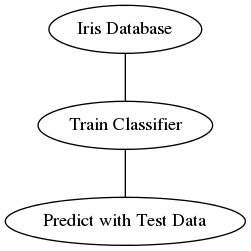You can replace any procedure in the process with a different one, perhaps better in some way, without compromising the whole system. For the model in the middle step, you can use an SVC or logistic regression: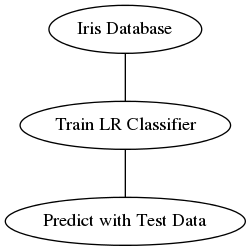One can also keep track of the classifier itself and build a flow diagram from the classifier. Here is a pipeline keeping track of the SVC classifier: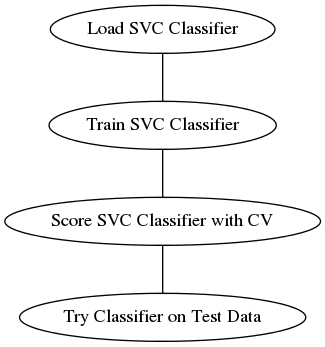In the upcoming chapters, we will see how scikit-learn uses the intuitive notion of a pipeline. So far, we have used a simple one: train, predict, test.

• Julian Avila

Julian Avila is a programmer and data scientist in finance and computer vision. He graduated from the Massachusetts Institute of Technology (MIT) in mathematics, where he researched quantum mechanical computation, a field involving physics, math, and computer science. While at MIT, Julian first picked up classical and flamenco guitars, Machine Learning, and artificial intelligence through discussions with friends in the CSAIL lab.

He started programming in middle school, including games and geometrically artistic animations. He competed successfully in math and programming and worked for several groups at MIT. Julian has written complete software projects in elegant Python with just-in-time compilation. Some memorable projects of his include a large-scale facial recognition system for videos with neural networks on GPUs, recognizing parts of neurons within pictures, and stock-market trading programs.

Browse publications by this author
• Trent Hauck

Trent Hauck is a data scientist living and working in the Seattle area. He grew up in Wichita, Kansas and received his undergraduate and graduate degrees from the University of Kansas. He is the author of the book Instant Data Intensive Apps with pandas How-to, Packt Publishing—a book that can get you up to speed quickly with pandas and other associated technologies.

Browse publications by this author
Latest Reviews (3 reviews total)
hassle free, thank you for you service
very cool 80% discount, I got a discount from a survey and bought the book right away
awesome book, very useful